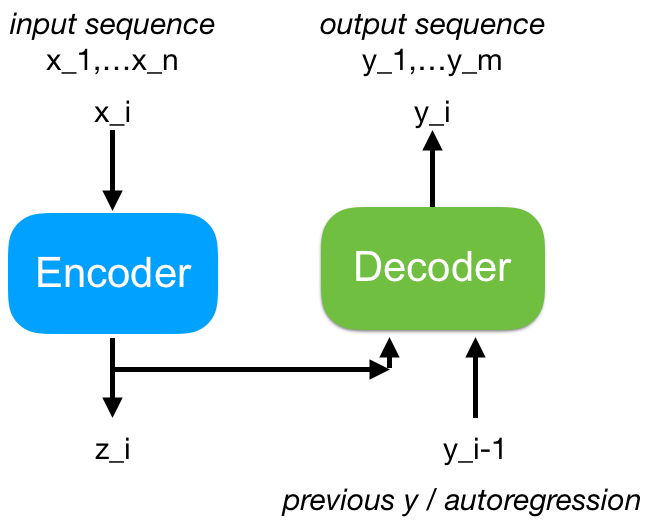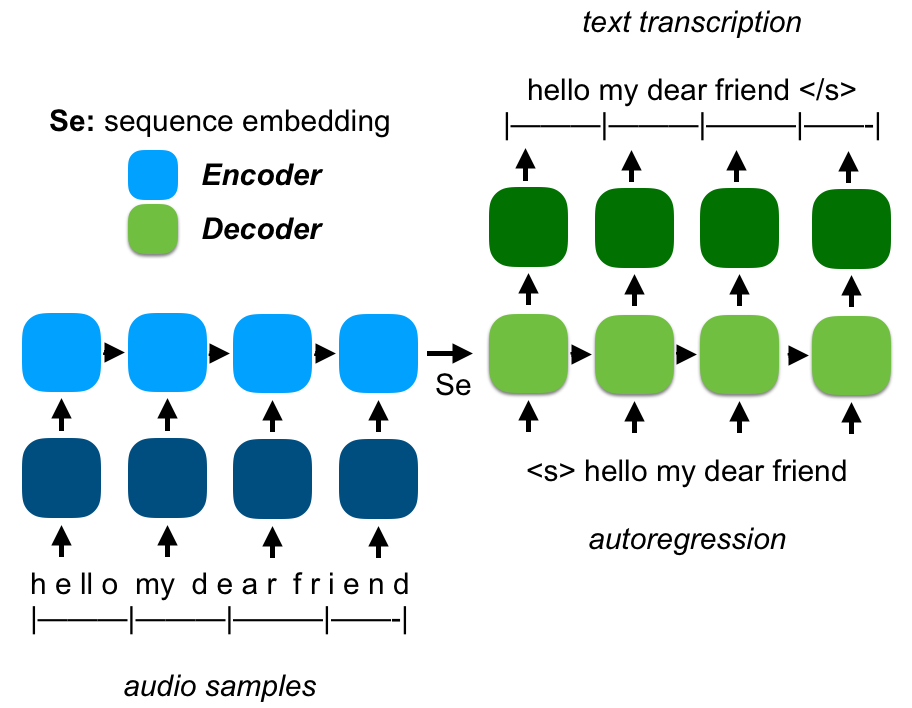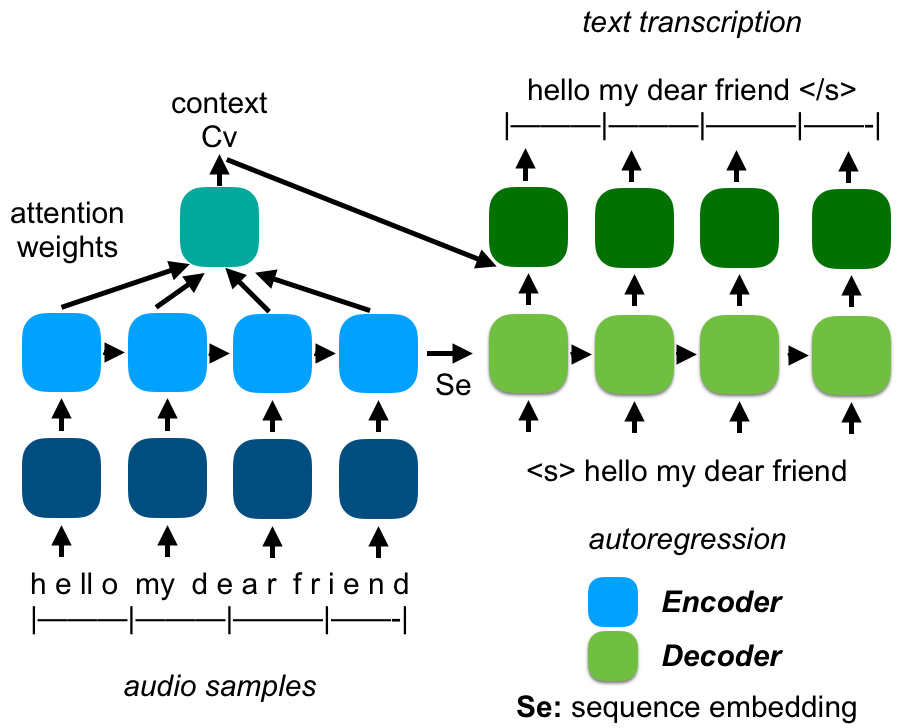Sequence-to-sequence neural networks

This posts explain the typical sequence-to-sequence (s2s) neural networks architecture and how to use them.

Introduction

Imagine we have in input sequence X= [x1, x2, x3, …] and an output sequence Y= [y1, y2, y3, y4, …]. Inputs and outputs respectively use sequences with input symbols and output symbols. These symbols can be different and often are.

Examples of s2s these are:

• language translation: inputs sequence in one language, output in another

s2s neural network are used to translate a sequence of symbols from one input sequence to an output sequence. These neural network usually use an encoder-decoder architecture (see figure below).Encoder-decoder neural network architecture for sequence-to-sequence learning. An input sequence Xi is encoded into a sequence vector Zi by the encoder. The decoder produces an output sequence Yi which is auto-regressive.

The encoder takes an input sequence x_i and encodes a long sequence of symbols into a sequence vector z_i. The decoder takes as input the sequence vector from the encoder and produces the output sequence y_i. The s2s decoder is generally auto-regressive, meaning that its outputs are fed back as decoder inputs at the next time step.

The encoder can take the entire sequence at once, or one input symbol at a time, and produce a sequence vector. This is a neural embedding for the entire input sequence. The encoder does not need to produce an output until the end-of-sequence </s> input symbol is received, then it does need to produce the output: the sequence vector.

The decoder can generate a sequence at once or one output symbol at a time.

Recurrent neural network s2s

The most typical neural network architecture used for sequence learning is the RNN / recurrent neural network. See also our blog post here. Thus it is only natural that the most common s2s neural network operates using RNNs.

A typical s2s RNN neural network still uses an encoder and a decoder, but they are both RNN networks. Typically they are LSTM or GRU-based neural networks with multiple layers. An example is in the figure below.s2s neural network based on RNN encoder and decoder. An inputs sequence is input one symbol at a time to the encoder RNN network (blue) to produce a sequence vector Se. The decoder is auto-regressive and takes the previous decoder output and the Se to produce one output symbol at a time.

The RNN encoder in the figure has two layer, one layer is an embedding layer, that takes inputs and translates them to a fixed code vector. This is called input embedding. The encoder produces a sequence vector Se. The decoder takes the vector Se and previous decoder output embedding to produce one output symbol at a time. The decoder in the figure has two layers, lighter green for the RNN network and darker green for the output network.

Note on figure: the input sequence here is much smaller than the real audio files one which can have several 10s of thousands of input samples.

Attention

A variation of the previous RNN encoder-decoder s2s network uses neural attention (see our blog post here) to modulate parts of the input sequence as we produce the output sequence.

Neural attention is of particular interest and efficacy in s2s network because as we translate one sequence from input to outputs not all input symbols are always important for each output symbols!

Imagine for example we want to translate the sentence: “I am going to the bank to process a check”. When we want to translate the word “bank” in another language, we need to focus on the input words: “… going … bank … check” because these input words will disambiguate the word “bank” as a “financial institution” and not a “heap” or “slope”, “mass” or “aircraft maneuver”.Neural attention is uses in s2s neural network to perform a weighted learned average of the input embeddings, so that the most accurate set of input symbols can be used to produce the proper output symbol.

As you can see from the figure above, the neural attention module takes inout from all embeddings of the input symbols, and the mixes them via a weighted average to a “context vector” Cv. This is then used to define which symbols are produced as output sequence.

Conclusions

In summary we looked at s2s neural network architecture for sequence processing, and the use of neural attention to focus on input symbols to produce more relevant output symbols.

For more information on this topic, please see our previous blog post which explains memory, sequences and attention neural networks.